KDnuggets Home » News » 2018 » Oct » Tutorials, Overviews » Preprocessing for Deep Learning: From covariance matrix to image whitening ( 18:n39 )

# Preprocessing for Deep Learning: From covariance matrix to image whitening

The goal of this post/notebook is to go from the basics of data preprocessing to modern techniques used in deep learning. My point is that we can use code (Python/Numpy etc.) to better understand abstract mathematical notions!comments

### 3. Image whitening

We will see how whitening can be applied to preprocess an image dataset. To do so we will use the paper of Pal & Sudeep (2016) where they give some details about the process. This preprocessing technique is called Zero component analysis (ZCA).

Check out the paper, but here is the kind of result they got. The original images (left) and the images after the ZCA (right) are shown.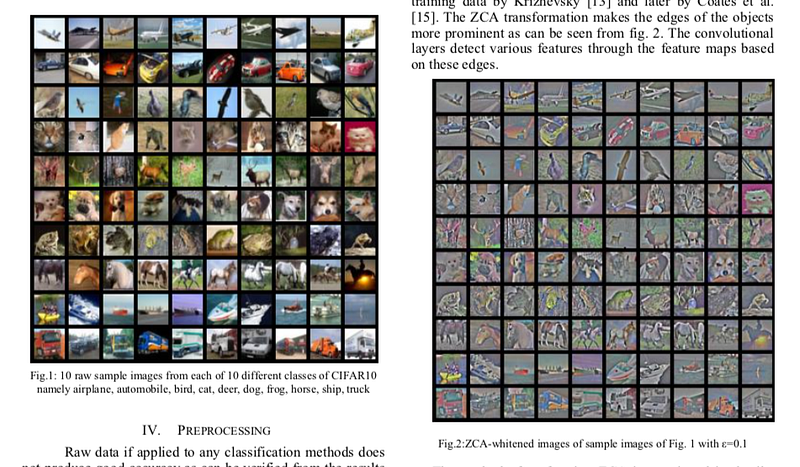Whitening images from the CIFAR10 dataset. Results from the paper of Pal & Sudeep (2016).

First things first. We will load images from the CIFAR dataset. This dataset is available from Keras and you can also download it here.

`(50000, 32, 32, 3)`

The training set of the CIFAR10 dataset contains 50000 images. The shape of `X_train` is `(50000, 32, 32, 3)`. Each image is 32px by 32px and each pixel contains 3 dimensions (R, G, B). Each value is the brightness of the corresponding color between 0 and 255.

We will start by selecting only a subset of the images, let’s say 1000:

`(1000, 32, 32, 3)`

That’s better. Now we will reshape the array to have flat image data with one image per row. Each image will be `(1, 3072)` because 32 x 32 x 3 = 3072. Thus, the array containing all images will be `(1000, 3072)`:

`(1000, 3072)`

The next step is to be able to see the images. The function `imshow()` from Matplotlib (doc) can be used to show images. It needs images with the shape (M x N x 3) so let’s create a function to reshape the images and be able to visualize them from the shape `(1, 3072)`.

For instance, let’s plot one of the images we have loaded: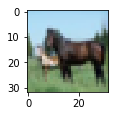Cute!
We can now implement the whitening of the images. Pal & Sudeep (2016)describe the process:

1. The first step is to rescale the images to obtain the range [0, 1] by dividing by 255 (the maximum value of the pixels).

Recall that the formula to obtain the range [0, 1] is:but, here, the minimum value is 0, so this leads to:```X.min() 0.0
X.max() 1.0```

Mean subtraction: per-pixel or per-image?

Ok cool, the range of our pixel values is between 0 and 1 now. The next step is:

2. Subtract the mean from all images.

Be careful here.

One way to do it is to take each image and remove the mean of this image from every pixel (Jarrett et al., 2009). The intuition behind this process is that it centers the pixels of each image around 0.

Another way to do it is to take each of the 3072 pixels that we have (32 by 32 pixels for R, G and B) for every image and subtract the mean of that pixel across all images. This is called per-pixel mean subtraction. This time, each pixel will be centered around 0 according to all images. When you will feed your network with the images, each pixel is considered as a different feature. With the per-pixel mean subtraction, we have centered each feature (pixel) around 0. This technique is commonly used (e.g Wan et al., 2013).

We will now do the per-pixel mean subtraction from our 1000 images. Our data are organized with these dimensions `(images, pixels)`. It was `(1000, 3072)` because there are 1000 images with 32 x 32 x 3 = 3072 pixels. The mean per-pixel can thus be obtained from the first axis:

`(3072,)`

This gives us 3072 values which is the number of means — one per pixel. Let’s see the kind of values we have:

```array([ 0.5234 , 0.54323137, 0.5274 , …, 0.50369804,
0.50011765, 0.45227451])```

This is near 0.5 because we already have normalized to the range [0, 1]. However, we still need to remove the mean from each pixel:

Just to convince ourselves that it worked, we will compute the mean of the first pixel. Let’s hope that it is 0.

```array([ -5.30575583e-16, -5.98021632e-16, -4.23439062e-16, …,
-1.81965554e-16, -2.49800181e-16, 3.98570066e-17])```

This is not exactly 0 but it is small enough that we can consider that it worked!

Now we want to calculate the covariance matrix of the zero-centered data. Like we have seen above, we can calculate it with the `np.cov()` function from NumPy.

Please note that our variables are our different images. This implies that the variables are the rows of the matrix X. Just to be clear, we will tell this information to NumPy with the parameter `rowvar=TRUE` even if it is `True` by default (see the doc):

Now the magic part — we will calculate the singular values and vectors of the covariance matrix and use them to rotate our dataset. Have a look at my poston the singular value decomposition (SVD)if you need more details.

Note: It can take a bit of time with a lot of images and that’s why we are using only 1000. In the paper, they used 10000 images. Feel free to compare the results according to how many images you are using:

In the paper, they used the following equation:with U the left singular vectors and S the singular values of the covariance of the initial normalized dataset of images, and X the normalized dataset. ϵ is an hyper-parameter called the whitening coefficient. diag(a) corresponds to a matrix with the vector a as a diagonal and 0 in all other cells.

We will try to implement this equation. Let’s start by checking the dimensions of the SVD:

`(1000, 1000) (1000,)`

S is a vector containing 1000 elements (the singular values). diag(S) will thus be of shape `(1000, 1000)` with S as the diagonal:

```[[ 8.15846654e+00 0.00000000e+00 0.00000000e+00 …, 0.00000000e+00
0.00000000e+00 0.00000000e+00]
[ 0.00000000e+00 4.68234845e+00 0.00000000e+00 …, 0.00000000e+00
0.00000000e+00 0.00000000e+00]
[ 0.00000000e+00 0.00000000e+00 2.41075267e+00 …, 0.00000000e+00
0.00000000e+00 0.00000000e+00]
…,
[ 0.00000000e+00 0.00000000e+00 0.00000000e+00 …, 3.92727365e-05
0.00000000e+00 0.00000000e+00]
[ 0.00000000e+00 0.00000000e+00 0.00000000e+00 …, 0.00000000e+00
3.52614473e-05 0.00000000e+00]
[ 0.00000000e+00 0.00000000e+00 0.00000000e+00 …, 0.00000000e+00
0.00000000e+00 1.35907202e-15]]

shape: (1000, 1000)```

Check this part:This is also of shape `(1000, 1000)` as well as U and U^T. We have seen also that X has the shape `(1000, 3072)`. The shape of X_ZCA is thus: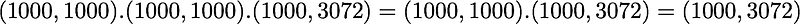which corresponds to the shape of the initial dataset. Nice.

We have: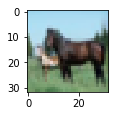Disappointing! If you look at the paper, this is not the kind of result they show. Actually, this is because we have not rescaled the pixels and there are negative values. To do that, we can put it back in the range [0, 1] with the same technique as above:

```min: 0.0
max: 1.0```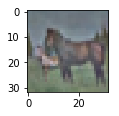Hooray! That’s great! It looks like an image from the paper. As mentioned earlier, they used 10000 images and not 1000 like us.

To see the differences in the results according to the number of images that you use and the effect of the hyper-parameter ϵ, here are the results for different values: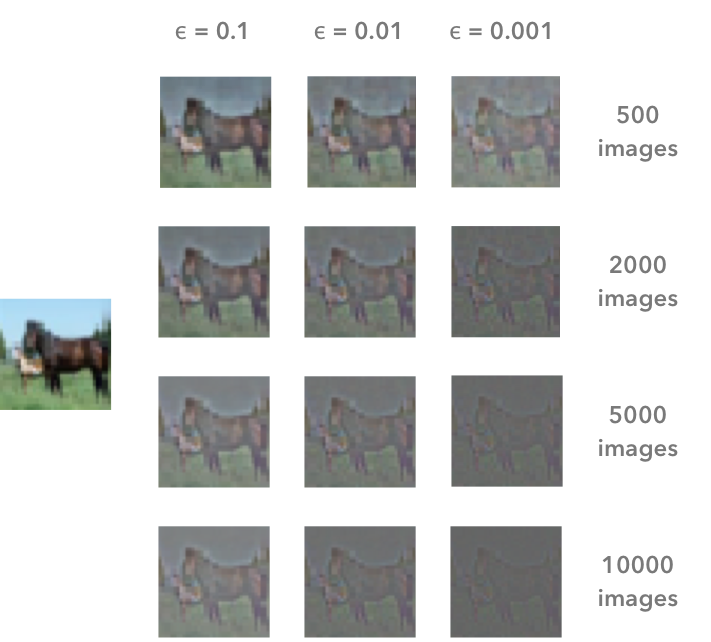The result of the whitening is different according to the number of images that we are using and the value of the hyper-parameter ϵ. The image on the left is the original image. In the paper, Pal & Sudeep (2016) used 10000 images and epsilon = 0.1. This corresponds to the bottom left image.

That’s all!

I hope that you found something interesting in this article You can read it on my blog, with LaTeX for the math, along with other articles.

You can also fork the Jupyter notebook on Github here.

References

K. Jarrett, K. Kavukcuoglu, M. Ranzato, and Y. LeCun, “What is the best multi-stage architecture for object recognition?,” in 2009 IEEE 12th International Conference on Computer Vision, 2009, pp. 2146–2153.

A. Krizhevsky, “Learning Multiple Layers of Features from Tiny Images,” Master’s thesis, University of Tront, 2009.

Y. A. LeCun, L. Bottou, G. B. Orr, and K.-R. Müller, “Efficient BackProp,” in Neural Networks: Tricks of the Trade, Springer, Berlin, Heidelberg, 2012, pp. 9–48.

K. K. Pal and K. S. Sudeep, “Preprocessing for image classification by convolutional neural networks,” in 2016 IEEE International Conference on Recent Trends in Electronics, Information Communication Technology (RTEICT), 2016, pp. 1778–1781.

L. Wan, M. Zeiler, S. Zhang, Y. L. Cun, and R. Fergus, “Regularization of Neural Networks using DropConnect,” in International Conference on Machine Learning, 2013, pp. 1058–1066.

Great resources and QA

Wikipedia — Whitening transformation

CS231 — Convolutional Neural Networks for Visual Recognition

Dustin Stansbury — The Clever Machine

Some details about the covariance matrix

SO — Image whitening in Python

Mean normalization per image or from the entire dataset

Mean subtraction — all images or per image?

Why centering is important — See section 4.3

Kaggle kernel on ZCA

How ZCA is implemented in Keras

Bio: Hadrien Jean is currently a PhD student in cognitive science (end expected: 2018) at the Ecole normale Supérieure (ENS) in Paris. He is studying auditory perceptual learning (pitch perception and auditory selective attention) using psychophysics and electrophysiology (EEG). During his PhD, he designed online auditory experiments using Django/Javascript. Passionate about data analysis, he used R to analyse behavioural data and create vizualisations and Python to analyse EEG data and elaborate offline and online signal processing workflows.

Original. Reposted with permission.

Related:

## Top Stories Past 30 DaysGet KDnuggets, a leading newsletter on AI, Data Science, and Machine Learning